# Compiler Design - Parser

In the previous chapter, we understood the basic concepts involved in parsing. In this chapter, we will learn the various types of parser construction methods available.

Parsing can be defined as top-down or bottom-up based on how the parse-tree is constructed.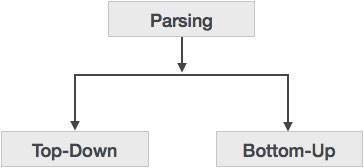## Top-Down Parsing

We have learnt in the last chapter that the top-down parsing technique parses the input, and starts constructing a parse tree from the root node gradually moving down to the leaf nodes. The types of top-down parsing are depicted below: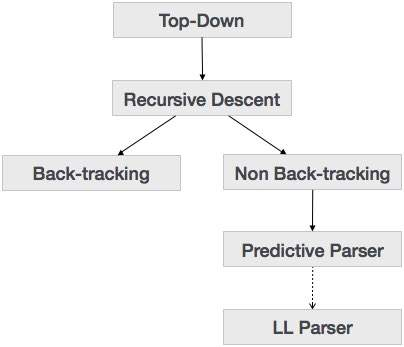### Recursive Descent Parsing

Recursive descent is a top-down parsing technique that constructs the parse tree from the top and the input is read from left to right. It uses procedures for every terminal and non-terminal entity. This parsing technique recursively parses the input to make a parse tree, which may or may not require back-tracking. But the grammar associated with it (if not left factored) cannot avoid back-tracking. A form of recursive-descent parsing that does not require any back-tracking is known as predictive parsing.

This parsing technique is regarded recursive as it uses context-free grammar which is recursive in nature.

### Back-tracking

Top- down parsers start from the root node (start symbol) and match the input string against the production rules to replace them (if matched). To understand this, take the following example of CFG:

```S → rXd | rZd
X → oa | ea
Z → ai
```

For an input string: read, a top-down parser, will behave like this:

It will start with S from the production rules and will match its yield to the left-most letter of the input, i.e. ‘r’. The very production of S (S → rXd) matches with it. So the top-down parser advances to the next input letter (i.e. ‘e’). The parser tries to expand non-terminal ‘X’ and checks its production from the left (X → oa). It does not match with the next input symbol. So the top-down parser backtracks to obtain the next production rule of X, (X → ea).

Now the parser matches all the input letters in an ordered manner. The string is accepted.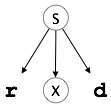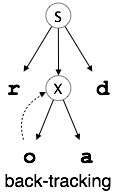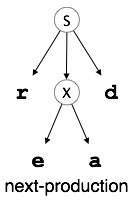### Predictive Parser

Predictive parser is a recursive descent parser, which has the capability to predict which production is to be used to replace the input string. The predictive parser does not suffer from backtracking.

To accomplish its tasks, the predictive parser uses a look-ahead pointer, which points to the next input symbols. To make the parser back-tracking free, the predictive parser puts some constraints on the grammar and accepts only a class of grammar known as LL(k) grammar.Predictive parsing uses a stack and a parsing table to parse the input and generate a parse tree. Both the stack and the input contains an end symbol \$ to denote that the stack is empty and the input is consumed. The parser refers to the parsing table to take any decision on the input and stack element combination.In recursive descent parsing, the parser may have more than one production to choose from for a single instance of input, whereas in predictive parser, each step has at most one production to choose. There might be instances where there is no production matching the input string, making the parsing procedure to fail.

### LL Parser

An LL Parser accepts LL grammar. LL grammar is a subset of context-free grammar but with some restrictions to get the simplified version, in order to achieve easy implementation. LL grammar can be implemented by means of both algorithms namely, recursive-descent or table-driven.

LL parser is denoted as LL(k). The first L in LL(k) is parsing the input from left to right, the second L in LL(k) stands for left-most derivation and k itself represents the number of look aheads. Generally k = 1, so LL(k) may also be written as LL(1).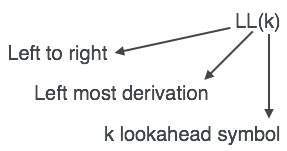### LL Parsing Algorithm

We may stick to deterministic LL(1) for parser explanation, as the size of table grows exponentially with the value of k. Secondly, if a given grammar is not LL(1), then usually, it is not LL(k), for any given k.

Given below is an algorithm for LL(1) Parsing:

```Input:
string ω
parsing table M for grammar G

Output:
If ω is in L(G) then left-most derivation of ω,
error otherwise.

Initial State : \$S on stack (with S being start symbol)
ω\$ in the input buffer

SET ip to point the first symbol of ω\$.

repeat
let X be the top stack symbol and a the symbol pointed by ip.

if X∈ Vt or \$
if X = a
else
error()
endif

else	/* X is non-terminal */
if M[X,a] = X → Y1, Y2,... Yk
POP X
PUSH Yk, Yk-1,... Y1 /* Y1 on top */
Output the production X → Y1, Y2,... Yk
else
error()
endif
endif
until X = \$	/* empty stack */
```

A grammar G is LL(1) if A → α | β are two distinct productions of G:

• for no terminal, both α and β derive strings beginning with a.

• at most one of α and β can derive empty string.

• if β → t, then α does not derive any string beginning with a terminal in FOLLOW(A).

## Bottom-up Parsing

Bottom-up parsing starts from the leaf nodes of a tree and works in upward direction till it reaches the root node. Here, we start from a sentence and then apply production rules in reverse manner in order to reach the start symbol. The image given below depicts the bottom-up parsers available.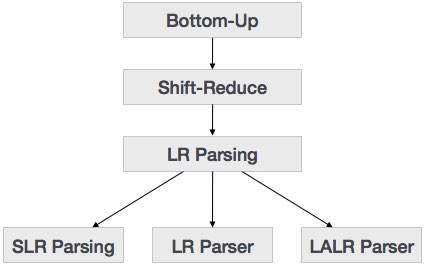### Shift-Reduce Parsing

Shift-reduce parsing uses two unique steps for bottom-up parsing. These steps are known as shift-step and reduce-step.

• Shift step: The shift step refers to the advancement of the input pointer to the next input symbol, which is called the shifted symbol. This symbol is pushed onto the stack. The shifted symbol is treated as a single node of the parse tree.

• Reduce step : When the parser finds a complete grammar rule (RHS) and replaces it to (LHS), it is known as reduce-step. This occurs when the top of the stack contains a handle. To reduce, a POP function is performed on the stack which pops off the handle and replaces it with LHS non-terminal symbol.

### LR Parser

The LR parser is a non-recursive, shift-reduce, bottom-up parser. It uses a wide class of context-free grammar which makes it the most efficient syntax analysis technique. LR parsers are also known as LR(k) parsers, where L stands for left-to-right scanning of the input stream; R stands for the construction of right-most derivation in reverse, and k denotes the number of lookahead symbols to make decisions.

There are three widely used algorithms available for constructing an LR parser:

• SLR(1) – Simple LR Parser:
• Works on smallest class of grammar
• Few number of states, hence very small table
• Simple and fast construction
• LR(1) – LR Parser:
• Works on complete set of LR(1) Grammar
• Generates large table and large number of states
• Slow construction
• LALR(1) – Look-Ahead LR Parser:
• Works on intermediate size of grammar
• Number of states are same as in SLR(1)

### LR Parsing Algorithm

Here we describe a skeleton algorithm of an LR parser:

```token = next_token()

repeat forever
s = top of stack

if action[s, token] = “shift si” then
PUSH token
PUSH si
token = next_token()

else if action[s, tpken] = “reduce A::= β“ then
POP 2 * |β| symbols
s = top of stack
PUSH A
PUSH goto[s,A]

else if action[s, token] = “accept” then
return

else
error()
```

### LL vs. LR

LL LR
Does a leftmost derivation. Does a rightmost derivation in reverse.
Starts with the root nonterminal on the stack. Ends with the root nonterminal on the stack.
Ends when the stack is empty. Starts with an empty stack.
Uses the stack for designating what is still to be expected. Uses the stack for designating what is already seen.
Builds the parse tree top-down. Builds the parse tree bottom-up.
Continuously pops a nonterminal off the stack, and pushes the corresponding right hand side. Tries to recognize a right hand side on the stack, pops it, and pushes the corresponding nonterminal.
Expands the non-terminals. Reduces the non-terminals.
Reads the terminals when it pops one off the stack. Reads the terminals while it pushes them on the stack.
Pre-order traversal of the parse tree. Post-order traversal of the parse tree.